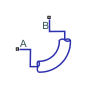# Pipe Bend (IL)

Pipe bend segment in an isothermal liquid network

Since R2020a

•Libraries:
Simscape / Fluids / Isothermal Liquid / Pipes & Fittings

## Description

The Pipe Bend (IL) block models a curved pipe in an isothermal liquid network. You can define the pipe characteristics to calculate losses due to friction and pipe curvature and optionally model fluid compressibility.

### Pipe Curvature Loss Coefficient

The coefficient for pressure losses due to geometry changes comprises an angle correction factor, Cangle, and a bend coefficient, Cbend:

`${K}_{loss}={C}_{angle}{C}_{bend}.$`

The block calculates Cangle as:

`${C}_{angle}=0.0148\theta -3.9716\cdot {10}^{-5}{\theta }^{2},$`

where θ is the value of the Bend angle parameter, in degrees.

The block calculates Cbend from the tabulated ratio of the bend radius, r, to the pipe diameter, d, for 90° bends from Crane :r/d11.5234681012141620
K20 fT14 fT12 fT12 fT14 fT17 fT24 fT30 fT34 fT38 fT42 fT50 fT

The bock interpolates the friction factor, fT, for clean commercial steel from tabular data based on the pipe diameter . This table contains the pipe friction data for clean commercial steel pipe with flow in the zone of complete turbulence.

Nominal size½"¾"1"1 ¼"1 ½"2"2 ½, 3"4"5"6"8-10"12-16"18-24"
Friction factor, fT.027.025.023.022.021.019.018.017.016.015.014.013.012

The correction factor is valid for a ratio of bend radius to diameter between 1 and 24. Beyond this range, the block employs nearest-neighbor extrapolation.

### Losses Due to Friction in Laminar Flows

The pressure loss formulations are the same for the flow at ports A and B.

When the flow in the pipe is fully laminar, or below Re = 2000, the pressure loss over the bend is:

`$\Delta {p}_{loss}=\frac{\mu \lambda }{2{\rho }_{I}{d}^{2}A}\frac{L}{2}{\stackrel{˙}{m}}_{port},$`

where:

• μ is the fluid dynamic viscosity.

• λ is the Darcy friction factor constant, which is 64 for laminar flow.

• ρI is the internal fluid density.

• d is the pipe diameter.

• L is the bend length segment, the product of the Bend radius and the Bend angle: ${L}_{bend}={r}_{bend}\theta .$.

• A is the pipe cross-sectional area, $\frac{\pi }{4}{d}^{2}.$

• ${\stackrel{˙}{m}}_{port}$ is the mass flow rate at the respective port.

### Losses due to Friction in Turbulent Flows

When the flow is fully turbulent, or greater than Re = 4000, the pressure loss in the pipe is:

`$\Delta {p}_{loss}=\left(\frac{{f}_{D}L}{2d}+\frac{{K}_{loss}}{2}\right)\frac{{\stackrel{˙}{m}}_{port}|{\stackrel{˙}{m}}_{port}|}{2{\rho }_{I}{A}^{2}},$`

where fD is the Darcy friction factor. This is approximated by the empirical Haaland equation and is based on the Internal surface absolute roughness. The differential is taken over half of the pipe segment, between port A to an internal node, and between the internal node and port B.

### Pressure Differential for Incompressible Fluids

When the flow is incompressible, the pressure loss over the bend is:

`${p}_{A}-{p}_{B}=\Delta {p}_{loss,A}-\Delta {p}_{loss,B}.$`

### Pressure Differential for Compressible Fluids

When the flow is compressible, the pressure loss over the bend is calculated based on the internal fluid volume pressure, pI:

`${p}_{A}-{p}_{I}=\Delta {p}_{loss,A}$`

`${p}_{B}-{p}_{I}=\Delta {p}_{loss,B}$`

### Mass Conservation

For an incompressible fluid, the mass flow into the pipe equals the mass flow out of the pipe:

`${\stackrel{˙}{m}}_{A}+{\stackrel{˙}{m}}_{B}=0.$`

When the fluid is compressible, the difference between the mass flow into and out of the pipe depends on the fluid density change due to compressibility:

`${\stackrel{˙}{m}}_{A}+{\stackrel{˙}{m}}_{B}={\stackrel{˙}{p}}_{I}\frac{d{\rho }_{I}}{d{p}_{I}}V,$`

where V is the product of the pipe cross-sectional area and bend length, AL.

## Ports

### Conserving

expand all

Liquid entry or exit port.

Liquid entry or exit port.

## Parameters

expand all

Diameter of the pipe.

Radius of the circle formed by the pipe bend.

Swept degree of the pipe bend.

Pipe wall absolute roughness. This parameter is used to determine the Darcy friction factor, which contributes to pressure loss in the pipe.

Whether to model any change in fluid mass due to fluid compressibility. When you select Fluid compressibility, mass changes due to varying fluid density in the segment are calculated. The fluid volume in the pipe remains constant. In the Isothermal Liquid library, all blocks calculate density as a function of pressure.

Pipe pressure at the beginning of the simulation.

#### Dependencies

To enable this parameter, select Fluid dynamic compressibility.

 Crane Co. Flow of Fluids Through Valves, Fittings, and Pipe TP-410. Crane Co., 1981.